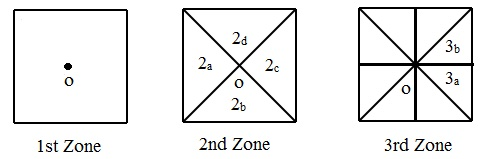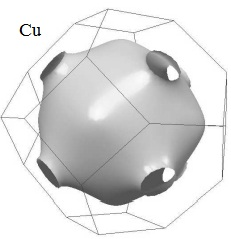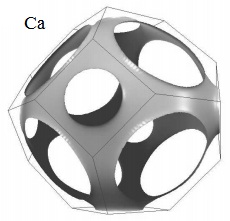#### Fermi Surfaces, Physics tutorial

Introduction:

The Fermi surface is basically the surface of constant energy εF in k space. The Fermi surface separates the unfilled orbital from the filled orbital, at absolute zero. The Quantum mechanics represented that the occupation of electron defines is governed through the Pauli exclusion and that the chemical potential, μ is equivalent to εF. The shape of the Fermi surface might be extremely intricate however the constructions needed the applications of the reduced and the periodic zone schemes. In the reduced zone scheme, it is for all time possible to choose the wave-vector index k of any Bloch function to lie in the first Brillouin zone. This method is termed as mapping the band in the reduced zone scheme. In the periodic zone, a given Brillouin zone is repeated periodically via all of the wave-vector space. This is accomplished through translating the zone via a reciprocal lattice.

Definition:

The Fermi energy surface is the energy distribution of particles which follow the Pauli Exclusion Principle.

Fermi surface:

The ground state of 'N' Bloch electrons is constructed in a similar fashion as that for free electrons, that is, by occupying all one-electron energy levels having band energies En(k) less than EF, where EF is found out by requiring the total number of levels having energies less than EF to be equivalent to the total number of electrons. The wave vector k should be confined to a single primitive cell of the reciprocal lattice. Whenever the lowest of such levels are filled through a specified number of electrons, two quite distinct kinds of configuration can result:

1) A specific number of bands might be fully filled, all others remaining empty. As the number of levels in a band is equivalent to the number of primitive cells in the crystal (and as each and every level can accommodate two electrons (that is, one of each spin), a configuration having a band gap can occur only if the number of electrons per primitive cell is even.

2) A number of bands might be partly filled. If this takes place, the energy of the highest occupied level, the Fermi energy EF, lies in the energy range of one or more bands. For each and every partially filled band there will be a surface in k-space separating the occupied from the unoccupied levels. The set of all these surfaces is termed as the Fermi surface, and is the generalization to Bloch electrons of the free electron Fermi sphere. The portions of the Fermi surface occurring from individual partially filled bands are termed as branches of the Fermi surface.

Analytically, the stream of the Fermi surface in the n-th band is that surface in k-space determined by:

En(k) = EF

Therefore the Fermi surface is a constant energy surface (or surfaces) in k-space.

As the En(k) are periodic in the reciprocal lattice, the complete solution to the above equation for each and every 'n' is a k-space surface having the periodicity of the reciprocal lattice. If a branch of the Fermi surface is symbolized by the full periodic structure, it is stated to be illustrated in a repeated zone scheme. Often, though, it is preferable to take just adequate of each and every branch of the Fermi surface so that each and every physically dissimilar level is symbolized by just one point of the surface. This is accomplished by representing each and every branch by that part of the full periodic surface contained in a single primitive cell of the reciprocal lattice. Such a representation is illustrated as a reduced zone scheme. The primitive cell selected is often, however not always, the first Brillouin zone.

Brillouin zones:

We now consider an illustration of building of a Fermi surface. We begin from considering the Fermi surface for free electrons and then examine the affect of the crystal potential. The Fermi surface for free electrons is a sphere centered at k = 0. To form the Fermi surface in the reduced-zone scheme, one can translate all the pieces of the sphere to the first zone via reciprocal lattice vectors. This method is made up of systematic via the geometrical notion of the higher Brillouin zones.We describe this construction for the (2-D) two dimensional cubic lattices as shown in figure above. Remember that the boundaries of the Brillouin zones are planes normal to 'G' at the midpoint of 'G'. The first Brillouin zone of the square lattice is the area enclosed through the perpendicular bisectors of G1 and of the three reciprocal lattice vectors equivalent via symmetry to G1 in the first part of the figure above. These four reciprocal lattice vectors are ± (2π/a)kxˆ and ± (2π/a)kyˆ

The second zone is made from G2 and the three vectors equivalent to it by symmetry, and likewise for the third zone. The pieces of the second and third zones are drawn in the second part of the figure above.

In common, the first Brillouin zone is the set of points in k-space which can be reached from the origin devoid of crossing any Bragg plane. The second Brillouin zone is the set of points which can be reached from the first zone by crossing just one Bragg plane. The (n+1)th Brillouin zone is the set of points not in the (n - l)th zone which can be reached from the nth zone by crossing only one Bragg plane.The free electron Fermi surface for a random electron concentration is represented in the figure shown above. Now we carry out a transformation to the reduced zone scheme as is illustrated in the figures shown below. We consider the triangle labeled 2a and move it through a reciprocal lattice vector G = (2π/a)kˆx in such a way that the triangle reappears in the area of the first Brillouin zone (Figure shown below). The other reciprocal lattice vectors will shift the triangles 2b, 2c, 2d to the other portions of the first zone, completing the mapping of the second zone to the reduced zone scheme. The portions of the Fermi surface falling in the second zone are now joined, as shown in the next figure shown below:The construction of Brillouin zones and Fermi surfaces in (3-D) three-dimensions is much more complex. The figure represents the first three Brillouin zones for bcc and fcc structures.Effect of a crystal potential:

How we can go from Fermi surfaces for free electrons to the Fermi surfaces in the presence of a weak crystal potential? We can form approximate constructions freehand via the use of the given facts:

1) The interaction of the electron by the periodic potential of the crystal causes the energy gaps at the zone boundaries.

2) Almost for all time the Fermi surface will intersect the zone boundaries perpendicularly. By using the equation for the energy close to the zone boundary it is simple to illustrate that (dE/dk) = [(h/2π)/m] (k - 1/2 G) that means that on the Bragg plane the gradient of energy is parallel to the Bragg plane. As the gradient is perpendicular to the surfaces on which the function is constant, the constant energy surfaces at the Bragg plane are perpendicular to the plane.

3) The crystal potential will round out sharp corners in the Fermi surfaces.

4) The net volume enclosed through the Fermi surface based only on the electron concentration and is independent of the details of the lattice interaction.

5) If a branch of the Fermi surface comprises of extremely small pieces of surface (that is, surrounding either occupied or unoccupied levels, termed as 'pockets of electrons' or 'pockets of holes'), then a weak periodic potential might cause these to disappear. Moreover, if the free electron Fermi surface consists of parts having a very narrow cross section, a weak periodic potential might cause it to become disconnected at these points.

Here are some of the illustrations for real metals.

Alkali metals:

The radius of Fermi sphere in bcc alkali metals is less than the minimum distance from the center of the zone to a zone face and thus the Fermi sphere lies completely within the first Brillouin zone. The crystal potential doesn't distort much the free electron Fermi surface and it remains extremely similar to a sphere.The noble metals:

The Fermi surface for a single half-filled free electron band in the fcc Bravais lattice is a sphere totally contained in the first Brillouin zone, approaching the surface of the zone most closely in the  directions, where it reaches 0.903 of the distance from the origin to the center of the hexagonal face. For all the three noble metals thus their Fermi surfaces are closely associated to the free electron sphere. Though, in the  directions contact is in reality made up by the zone faces, and the measured Fermi surfaces encompass the shape shown in figure given below. Eight 'necks' reach out to touch the eight hexagonal faces of the zone, however or else the surface is not grossly distorted from the spherical.The cubic divalent metals:

By two electrons per primitive cell, calcium, strontium and barium could, in principle, be insulators. In the free electron model, the Fermi sphere consists of the similar volume as the first zone and thus intersects the zone faces. The free electron Fermi surface is therefore a fairly complex structure in the first zone, and pockets of electrons in the second. The question is whether the effective lattice potential is strong adequate to shrink the second-zone pockets down to zero volume, thus filling up all the unoccupied levels in the first zone. Obviously this is not the condition, since the group II elements are all metals. Computations illustrate that the first Brillouin zone is fully filled and a small number of electrons in the second zone found out the non-zero conductance.Trivalent metals:

The Fermi surface of aluminum is close to that of the free electron surface for the fcc cubic monoatomic lattice having three conduction electrons per atom. The first Brillouin zone is filled and the Fermi surface of free electrons is completely contained in the second, third and fourth Brillouin zones. Whenever shown in a reduced-zone scheme the second-zone surface is a closed structure having unoccupied levels, whereas the third-zone surface is a complex structure of the narrow tubes. The amount of surface in the fourth zone is extremely small, enclosing small pockets of the occupied levels.

The effect of a weak periodic potential is to eradicate the fourth-zone pockets of electrons and decrease the third-zone surface to a set of the disconnected rings.Aluminum gives a striking description of the theory of Hall coefficients. The high-field Hall coefficient must be RH = - 1/(ne - nh ) e , where ne and nh are the number of levels per unit volume enclosed through the particle-like and hole-like branches of the Fermi surface. As the first zone of aluminum is fully filled and accommodates two electrons per atom, one of the three valence electrons per atom remains to occupy second and third-zone levels. Therefore:

neII + neIII = n/3

Here 'n' is the free electron carrier density suitable to valence 3. On the other hand, as the net number of levels in any zone is adequate to hold the two electrons per atom, we as well encompass:

neII + nhII = 2(n/3)

Subtracting both the above two equation, we get:

neIII - nhII = - (n/3)

Therefore the high-field Hall coefficient must encompass a positive sign and yield an effective density of carriers a third of the free electron value. This is exactly what is observed.

Tutorsglobe: A way to secure high grade in your curriculum (Online Tutoring)